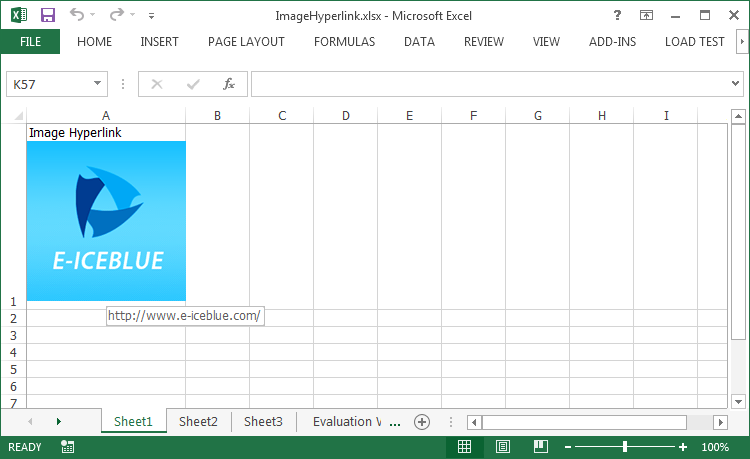Code Snippet:

Step 1: Create an object of Workbook and get the first worksheet.

```Workbook wb = new Workbook();
Worksheet sheet = wb.Worksheets;
```

Step 2: Insert an image to a specific cell.

```string picPath = @"C:\Users\Administrator\Desktop\logo.png";
ExcelPicture picture = sheet.Pictures.Add(1, 1, picPath);
```

```picture.SetHyperLink("Http://www.e-iceblue.com", true);
```

Step 4: Set the 1st column width and 1st row height.

```sheet.Columns.ColumnWidth = 22;
sheet.Rows.RowHeight = 140;
```

Step 5: Save the file.

```wb.SaveToFile("ImageHyperlink.xlsx", ExcelVersion.Version2013);
```

Output:Full Code:

[C#]
```Workbook wb = new Workbook();
Worksheet sheet = wb.Worksheets;

sheet.Range["A1"].Style.VerticalAlignment = VerticalAlignType.Top;

ExcelPicture picture = sheet.Pictures.Add(1, 1, picPath);

sheet.Columns.ColumnWidth = 22;
sheet.Rows.RowHeight = 140;
picture.TopRowOffset = 25;

```
[VB.NET]
```Dim wb As New Workbook()
Dim sheet As Worksheet = wb.Worksheets(0)